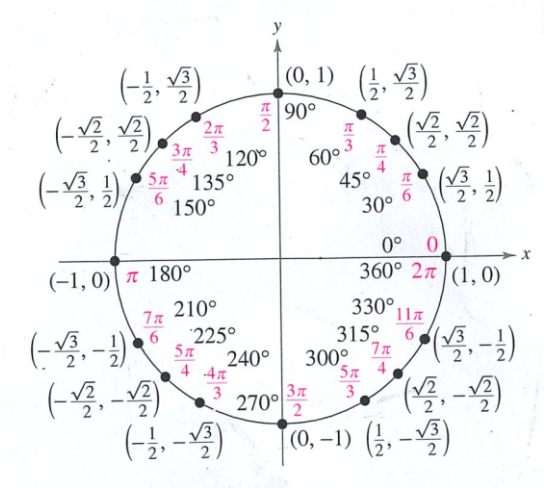Exit Ticket for Day 2 of Trig
Please select your name from this list: *
You can use the following diagram of the unit circle -- which I scanned from the front of your textbook -- on this Exit Ticket.The next six questions all refer to the angle π/3.
1. Using the above unit circle, evaluate sin(π/3).
2. Using the above unit circle, evaluate cos(π/3).
3. Using the above unit circle, evaluate tan(π/3).
4. Using the above unit circle, evaluate sec(π/3).
5. Using the above unit circle, evaluate csc(π/3).
6. Using the above unit circle, evaluate cot(π/3).
This form was created inside of Evansville Vanderburgh School Corporation. Report Abuse - Terms of Service